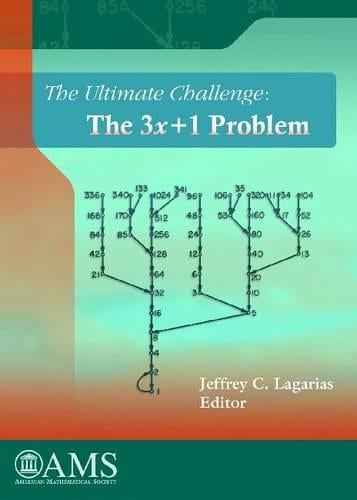•# The Ultimate Challenge: The 3x 1 Problem (Hardback)

(editor)
£59.95
Hardback 344 Pages / Published: 30/01/2011
• We can order this from the publisher

Usually dispatched within 10 working days

The $3x 1$ problem, or Collatz problem, concerns the following seemingly innocent arithmetic procedure applied to integers: If an integer $x$ is odd then ""multiply by three and add one"", while if it is even then ""divide by two"". The $3x 1$ problem asks whether, starting from any positive integer, repeating this procedure over and over will eventually reach the number 1. Despite its simple appearance, this problem is unsolved. Generalizations of the problem are known to be undecidable, and the problem itself is believed to be extraordinarily difficult. This book reports on what is known on this problem. It consists of a collection of papers, which can be read independently of each other. The book begins with two introductory papers, one giving an overview and current status, and the second giving history and basic results on the problem. These are followed by three survey papers on the problem, relating it to number theory and dynamical systems, to Markov chains and ergodic theory, and to logic and the theory of computation. The next paper presents results on probabilistic models for behavior of the iteration. This is followed by a paper giving the latest computational results on the problem, which verify its truth for $x < 5.4 \cdot 10^{18}$. The book also reprints six early papers on the problem and related questions, by L. Collatz, J. H. Conway, H. S. M. Coxeter, C. J. Everett, and R. K. Guy, each with editorial commentary. The book concludes with an annotated bibliography of work on the problem up to the year 2000.

Publisher: American Mathematical Society
ISBN: 9780821849408
Number of pages: 344
Weight: 778 g
Dimensions: 229 x 152 mm